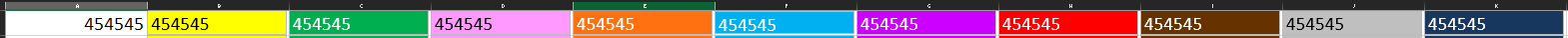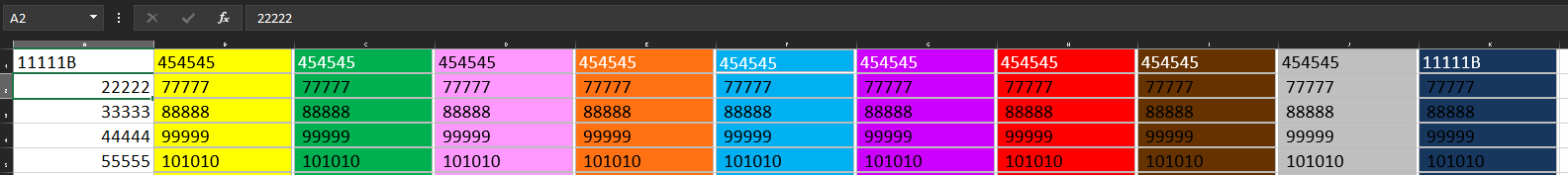asked on

# Creating Macro but cannot get vb code modified

I have created a macro that should copy the contents of cell A1 and then paste it into the 10 shapes.  What appears in the macro is it has stored a static value and copies the same text into the shapes no matter what I put in Cell A1.  Could someone help me get this working.  Feeling so defeated right now.  Below is the code from the macro.``````Sub CreateBlocks()
'
' CreateBlocks Macro
' CreateBlocks
'

'
Range("A1").Select
ActiveCell.FormulaR1C1 = "454545"
ActiveSheet.Shapes.Range(Array("TextBox 111")).Select
ActiveSheet.Shapes.Range(Array("TextBox 564")).Select
ActiveSheet.Shapes.Range(Array("TextBox 565")).Select
ActiveSheet.Shapes.Range(Array("TextBox 566")).Select
ActiveSheet.Shapes.Range(Array("TextBox 567")).Select
ActiveSheet.Shapes.Range(Array("TextBox 568")).Select
ActiveSheet.Shapes.Range(Array("TextBox 569")).Select
ActiveSheet.Shapes.Range(Array("TextBox 570")).Select
ActiveSheet.Shapes.Range(Array("TextBox 571")).Select
ActiveSheet.Shapes.Range(Array("TextBox 572")).Select
Selection.ShapeRange(1).TextFrame2.TextRange.Characters.Text = "454545"
Selection.ShapeRange(1).TextFrame2.TextRange.Characters(1, 6).ParagraphFormat. _
FirstLineIndent = 0
With Selection.ShapeRange(1).TextFrame2.TextRange.Characters(1, 6).Font
.NameComplexScript = "+mn-cs"
.NameFarEast = "+mn-ea"
.Fill.Visible = msoTrue
.Fill.ForeColor.ObjectThemeColor = msoThemeColorBackground1
.Fill.ForeColor.Brightness = 0
.Fill.Transparency = 0
.Fill.Solid
.Size = 36
.Name = "+mn-lt"
End With
Range("A2").Select
End Sub

``````
VB ScriptMicrosoft ExcelMicrosoft OfficeLast Comment
Trygve Thayer

8/22/2022 - Mon
Rob Henson

Dim Txt as String

Delete Line 9

Change:
Line 8:  Txt = Range("A1").Value
Line 20: Selection.ShapeRange(1).TextFrame2.TextRange.Characters.Text = Txt
Trygve Thayer

Made some progress as it did populate one shape on the sheet but the others did not change.  When we get this row working I plan to add to the macro to do additional rows.``````Sub CreateBlocks()
'
' CreateBlocks Macro
' CreateBlocks
'

'
Dim Txt As String
Txt = Range("A1").Value
ActiveSheet.Shapes.Range(Array("TextBox 111")).Select
ActiveSheet.Shapes.Range(Array("TextBox 564")).Select
ActiveSheet.Shapes.Range(Array("TextBox 565")).Select
ActiveSheet.Shapes.Range(Array("TextBox 566")).Select
ActiveSheet.Shapes.Range(Array("TextBox 567")).Select
ActiveSheet.Shapes.Range(Array("TextBox 568")).Select
ActiveSheet.Shapes.Range(Array("TextBox 569")).Select
ActiveSheet.Shapes.Range(Array("TextBox 570")).Select
ActiveSheet.Shapes.Range(Array("TextBox 571")).Select
ActiveSheet.Shapes.Range(Array("TextBox 572")).Select
Selection.ShapeRange(1).TextFrame2.TextRange.Characters.Text = Txt
Selection.ShapeRange(1).TextFrame2.TextRange.Characters(1, 6).ParagraphFormat. _
FirstLineIndent = 0
With Selection.ShapeRange(1).TextFrame2.TextRange.Characters(1, 6).Font
.NameComplexScript = "+mn-cs"
.NameFarEast = "+mn-ea"
.Fill.Visible = msoTrue
.Fill.ForeColor.ObjectThemeColor = msoThemeColorBackground1
.Fill.ForeColor.Brightness = 0
.Fill.Transparency = 0
.Fill.Solid
.Size = 36
.Name = "+mn-lt"
End With
Range("A2").Select
End Sub

``````
Trygve Thayer

Does anyone have additional comments?  Working through on my own and making progress but having problem with this line
Selection.ShapeRange(1).TextFrame2.TextRange.Characters(1, 32).ParagraphFormat. _
FirstLineIndent = 0

Is there any way I can just select al in the cell
Rob Henson

Couple of questions.

How come you're using lots of text box/shapes?
Any reason why it can't just go in a cell?

A sample file would be useful.
Norie

THIS SOLUTION ONLY AVAILABLE TO MEMBERS.
View this solution by signing up for a free trial.
Members can start a 7-Day free trial and enjoy unlimited access to the platform.
Trygve Thayer

Rob,

The reason I am doing it this way we are using an excel spreadsheet on a tab that has a template of a flatbed trailer.  On another tab we are copying and pasting the parts to but on the trailer from our erp system.  By putting them in shapes with the text of the part we can drag and place them where we want them positioned on the template as well as color code them to indicate what gets removed at each stop.

Trygve Thayer

Norie,

Worked Perfectly !!!!!!
Rob Henson

I assume each part will be a different size then.

I guess ideal scenario would be
1) a parts template sheet with dimensions and text for each stock item,
2) an input sheet to select Stock Item part number & order quantities,
3) a trailer template sheet.

Select part Numbers and quantities and then click a button to run a routine that creates the relevant number of shapes on the trailer sheet so that the user can re-arrange on that sheet for loading the trailer.
Trygve Thayer